# Binary Puzzles

As you can probably tell, I’m a big fan of puzzles. On one hand you can say that a good puzzle is nothing but particular instance of a complex problem that we’re being asked to solve. What exactly makes a problem complex though?

To a large extent that depends on the person playing the puzzles. Different puzzles are based on different concepts and meant to highlight different concepts. Some puzzles really focus on dynamic programming like the Triangle Sum Puzzles or the Unidirectional TSP Puzzles.

Other puzzles are based on more complicated problems, in many cases instances of NP-complete problems. Unlike the puzzles mentioned above, there is generally no known optimal strategy for solving these puzzles quickly. Some basic examples of these are ones like Independent Set Puzzles, which just give a random (small) instance of the problem and ask users to solve it. Most approaches involve simply using logical deduction to reduce the number of possible choices until a “guess” must be made and then implementing some form of backtracking solution (which is not guessing since you can form a logical conclusion that if the guess you made were true, you reach either (a) a violation of the rules or (b) a completed puzzle).

One day a few months back i was driving home from work and traffic was so bad that i decided to stop at the store. While browsing the books, I noticed a puzzle collection. Among the puzzles I found in that book were the Range Puzzles I posted about earlier. However I also found binary puzzles.

Filled Binary puzzles are based on three simple rules
1. No the adjacent cells in any row or column can contain the same value (so no 000 or 111 in any row or column).
2. Every row must have the same number of zeros and ones.
3. Each row and column must be unique.

There is a paper from 2013 stating that Binary Puzzles are NP Complete. There is another paper that discusses strategies involved in Solving a Binary Puzzle

Once I finished the puzzles in that book the question quickly became (as it always does) where can I get more. I began writing a generator for these puzzles and finished it earlier this year. Now i want to share it with you. You can visit the examples section to play those games at Binary Puzzles.

Below I will go over a sample puzzle and how I go about solving it. First lets look at a 6 by 6 puzzle with some hints given:

 0 1 0 1 0 1 1 0 1 0 0 1 1 0

We look at this table and can first look for locations where we have a “forced move”. An obvious choice for these moves wold be three adjacent cells in the same row or column where two have the same value. A second choice is that when we see that a row or column has the correct number of zeros or ones, the remaining cells in that row or column must have the opposite value.

So in the above puzzle, we can see that the value in cells (2, 2) and (2, 5) must also be a 0 because cells (2, 3) and (2, 4) are both 1. Now we see that column 2 has 5 of its 6 necessary values, and three 0’s. So the last value in this column (2, 6) must be a 1 in order for there to be an equal number of 0s and 1s.

For some easier puzzles these first two move types will get you far enough to completely fill in all the cells. For more advanced puzzles though, this may require a little more thorough analysis.

As always, check it out and let me know what you think.

# Discrete-time Markov Chains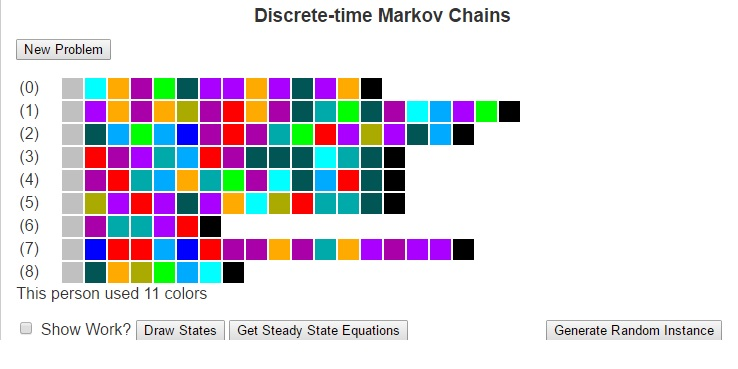Much of how we interact with life could be described as transitions between states. These states could be weather conditions (whether we are in a state of “sunny” or “rainy”), the places we may visit (maybe “school”, “the mall”, “the park” and “home”), our moods (“happy”, “angry”, “sad”). There are a number of other ways to model states and even the possibility of infinitely many states.

Markov Chains are based on the principle that the future is only dependent on the immediate past. so for example, if I wished to predict tomorrow’s weather using a Markov Chain, I would need to only look at the weather for today, and can ignore all previous data. I would then compare the state of weather for today with historically how weather has changed in between states to determine the most likely next state (i.e what the weather will be like tomorrow). This greatly simplifies the construction of models.

To use Markov Chains to predict the future, we first need to compute a transition matrix which shows the probability (or frequency) that we will travel from one state to another based on how often we have done so historically. This transition matrix can be calculated by looking at each element of the history as an instance of a discrete state, counting the number of times each transition occurs and dividing each result by the number of times the origin state occurs. I’ll next give an example and then I’ll focus on explaining the Finite Discrete State Markov Chain tool I built using javascript.

Next, I want to consider an example of using Markov Chains to predict the weather for tomorrow. Suppose that we have observed the weather for the last two weeks. We could then use that data to build a model to predict tomorrow’s weather. To do this, lets first consider some states of weather. Suppose that a day can be classified in one of four different ways: {Sunny, Cloudy, Windy, Rainy}. Further, suppose that over the last two weeks we have seen the following pattern.

 Day 1 Sunny Day 2 Sunny Day 3 Cloudy Day 4 Rain Day 5 Sunny Day 6 Windy Day 7 Rain Day 8 Windy Day 9 Rain Day 10 Cloudy Day 11 Windy Day 12 Windy Day 13 Windy Day 14 Cloudy

We can look at this data and calculate the probability that we will transition from each state to each other state, which we see below:

 Rain Cloudy Windy Sunny Rain 0 1/3 1/3 1/3 Cloudy 1/2 0 1/2 0 Windy 2/5 1/5 2/5 0 Sunny 0 1/3 1/3 1/3

Given that the weather for today is cloudy, we can look at the transition matrix and see that historically the days that followed a cloudy day have been Rainy and Windy days each with probability of 1/5. We can see this more mathematically by multiplying the current state vector (cloudy) [0, 1, 0, 0] by the above matrix, where we obtain the result [1/2, 0, 1/2, 0].

In similar fashion, we could use this transition matrix (lets call it T) to predict the weather a number of days in the future by looking at Tn. For example, if we wanted to predict the weather two days in the future, we could begin with the state vector [1/2, 0, 1/2, 0] and multiply it by the matrix T to obtain [1/5, 4/15, 11/30, 1/6].

We can also obtain this by looknig at the original state vector [0, 1, 0, 0] and multiplying it by T2.

T2 =

1

 3/10 8/45 37/90 1/9 1/5 4/15 11/30 1/6 13/50 16/75 59/150 2/15 3/10 8/45 37/90 1/9

When we multiply the original state vector by T2 we arrive at this same answer [1/5, 4/15, 11/30, 1/6]. This matrix T2 has an important property in that every state can reach every other state.

In general, if we have a transition matrix where for every cell in row i and column j, there is some power of the transition matrix such that the cell (i, j) in that matrx is nonzero, then we say that every state is reachable from every other state and we call the Markov Chain regular.

Regular Markov Chains are important because they converge to what’s called a steady state. These are state vectrs x = [x0, …, xn] such that xTn = x for very large values of n. The steady state tells us how the Markov Chain will perform over long periods of time. We can use algebra and systems of linear equations to solve for this steady state vector.

For the Javascript program I’ve written, I have generated a set of painting samples for a fictional artist. The states are the different colors and the transitions are the colors that the artist will use after other colors. as well as the starting and ending colors. Given this input, we can form a Markov Chain to understand the artist’s behavior. This Markov Chain can then be used to solve for the steady state vector or to generate random paintings according to the artist’s profile. Be sure to check it out and let me know what you think.

# The RSA Algorithm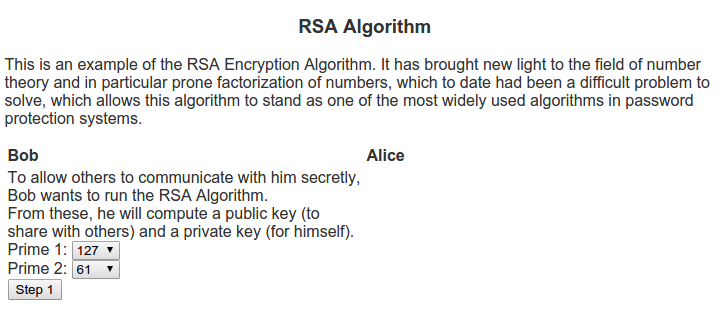I can remember back when I was in school, still deciding whether I wanted to study pure or applied mathematics. One of the common questions I would receive from those in applied mathematical realms would sound like “What’s the point of doing mathematics with no real world applications?”. Generally my response to these questions was about the intrinsic beauty of mathematics, no different from an artist painting not for some desire to be a millionaire, but because of an burning desire to paint. Whether their paintings would one day be on the walls of a Smithsonian museum or sit on their mother’s refrigerator is generally outside of the thought process of the artist. So too, would I argue about the thought process of a pure mathematician.

When I was an undergrad and learned about the RSA algorithm (named for Ron Rivest, Adi Shamir, and Leonard Adleman who discovered the algorithm) it helped me explain this concept a lot better. The algorithm is based on prime numbers and the problem of finding the divisors of a given number. Many mathematicians throughout the ages have written papers on the beauty of prime numbers (see Euclid, Eratosthenes, Fermat, Goldbach, etc). For a large period in time one of the beautiful things about prime numbers was that they were so interesting in themselves. There were questions about how to check if a number is prime, question of patterns in primes, famous conjectures like the Goldbach conjecture and the twin prime conjecture, quick ways of finding prime numbers or numbers that are almost always prime, etc. In short, this was an active area of research that much of the applied world was not using. This all changed in 1977 when Rivest, Shamir and Adleman published the RSA algorithm.

The algorithm is in the area called public key cryptography. These algorithms differ from many of the previous cryptography algorithms, namely symmetric key cryptography. Whereas symmetric key cryptography depends uses the same device (key) to encode as to decode, public key cryptography creates two keys – one for encoding that is generally shared with others, and another for decoding which is kept private. These two keys in generally relate to a mathematical problem that is very difficult to solve.

In my example script for the RSA Algorithm, I show two people who want to communicate, Alice and Bob. Bob wants people to be able to send him messages securely so he decides to use the RSA algorithm. To do this, he first needs to think of two prime numbers, p1 and p2.
From these, he computes the following:
n = p1 * p2

Next, he computes Euler’s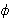function on this n which can be calculated as(n) = (p1 – 1) * (p2 – 1)

Then Bob looks for a number e that is relatively prime to. This is what he will use as the encryption key.

One he has e, he can calculate d, which is the multiplicative inverse of e in
(n).
This means that e * d = 1 (mod(n)).

The public key that will be used for encryption will be the pair (e, n). This is what he posts publicly via his web page for others to communicate with him securely. Bob also has a private key pair (d, n) that he will use to decrypt messages.

Alice sees Bob’s public key and would like to communicate with him. So she uses it to encode a message. The formula she uses to encrypt her message is c = me mod n, where c is the encrypted message. Once Alice encrypts her message, she sends it to Bob.

Bob receives this encoded message and uses the private key (d, n) to decode the message from Alice. The formula to decrypt is m = cd mod n.

For a more illustrative idea of how this algorithm works as well as examples, be sure to visit Script for the RSA Algorithm.

# QR DecompositionSuppose we have a problem that can be modeled by the system of equations Ax = b with a matrix A and a vector b. We have already shown how to use Gaussian Elimination to solve these methods, but I would like to introduce you to another method – QR decomposition. In addition to solving the general system of equations, this method can also be used in a number of other algorithms including the linear regression analysis to solve the least squares problem and to find the eigenvalues of a matrix.

Generally, when we see a system of equations, the best way to proceed in solving it is Gaussian elimination, or LU decomposition. However, there are some very special matrices where this method can lead to rounding errors. In such cases, we need a better, or more stable algorithm, which is when the QR decomposition method becomes important.

Any matrix A, of dimensions m by n with m >= n, can be represented as the product of two matrices, an m by m orthogonal matrix Q, i.e. QTQ = I, and an m by n upper triangular matrix R with the form [R 0]T. We perform this decomposition by will first converting the columns of the matrix A into vectors. Then, for each vector ak, we will calculate the vectors uk and ek given by

uk =i = 1 to k-1projej ak
and
ek = uk / ||uk||
Then Q = [e1, …, en] and

R =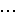00 0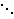# Introduction to JavaScript Programming

I received a lot of attention from friends interested in programming after my recent blog post entitled “Introduction to Python Programming”. While many found it interesting, the fact that Python is more useful to mathematicians hindered sine of my friends desire to learn it as their first language.

In out conversations, my recommendation for a first language was JavaScript. This is a powerful language in the sense that just about anybody who is involved with the internet knows it, and it’s likely to boost a person’s resume. It also has many similarities to more powerful languages like C++ and Java, so while not trivial, it could be a good launch pad into more advanced languages. But my favorite reason is that unlike many other programming languages that rely in an MS-DOS like command like approach for run time interaction, JavaScript’s basic interaction is with the standard internet browsers we use everyday. There isn’t even anything you need to download or install. Just create a basic HTML file in a text editor (like notepad, wordpad, or notepad++). This makes it easier to show off your creations which makes learning more fun.

The script I’ve finished provides examples on writing output, declaring variables, data types, conditionals, loops, and functions. Although I do not go into detail about all the events and objects on an HTML page, I do finish with three examples of more advanced JavaScript programs. Once you’ve selected a program, the code well be revealed in the text area. There is also a button that, when clicked, will execute that script on a new HTML tab.

I hope you enjoy, and let me know if you have any suggestions or comments.

With that being said, here is a link to my sample JavaScript code.